## How to Calculate the Volume, Length, Width and Height of a Cuboid | Nickzom Calculator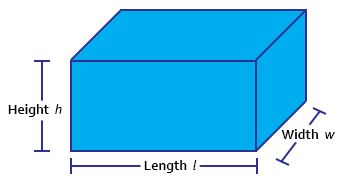The image above represents a cuboid.
To compute the volume of a cuboid requires three essential parameters which are the length, width and height of the cuboid.

The formula for computing the volume of a cuboid is:

V = lwh

Where:
V = Volume of the Cuboid
l = Length of the Cuboid
w = Width of the Cuboid
h = Height of the Cuboid

Let’s solve an example
Find the volume of a cuboid with a length of 6 cm, width of 2 cm and a height of 10 cm.

This implies that:
l = length of the cuboid = 6
w = width of the cuboid = 2
h= = height of the cuboid = 10

V = lwh
V = 6 x 2 x 10
V = 120

Therefore, the volume of the cuboid is 120 cm3.

Calculating the Length of a cuboid when Volume, Width and Height are Given

The formula is l = V / (w)(h)

Where;
V = Volume of the Cuboid
l = Length of the Cuboid
w = Width of the Cuboid
h = Height of the Cuboid

Let’s solve an example:
Find the length of a cuboid with a volume of 440 cm3 , a width of 5 cm and a height of 11 cm.

This implies that;
V = Volume of the cuboid = 440 cm3
w = width of the cuboid = 5 cm
h  = height of the cuboid = 11 cm

l =  V / (w)(h)
l = 440 / (5)(11)
l = 440 / 55
l = 8 cm

Therefore, the length of the cuboid is 8 cm.

Calculating the Width of a cuboid when Volume, Length and Height are Given

The formula is w = V / (l)(h)

Where;
V = Volume of the Cuboid
l = Length of the Cuboid
w = Width of the Cuboid
h = Height of the Cuboid

Let’s solve an example:
Find the width of a cuboid with a volume of  180 cm3 , a length of 6 cm and a height of 10 cm

This implies that;
V = Volume of the cuboid = 180 cm3
l = length of the cuboid = 6 cm
h  = height of the cuboid = 10 cm

w =  V / (l)(h)
w = 180 / (6)(10)
w = 180 / 60
w = 3 cm

Therefore, the width of the cuboid is 3 cm.

Calculating the Height of a cuboid when Volume, Length and Width are Given

The formula is h = V / (l)(w)

Where;
V = Volume of the Cuboid
l = Length of the Cuboid
w = Width of the Cuboid
h = Height of the Cuboid

Let’s solve an example:
Find the height of a cuboid with a volume of 195 cm3 , a length of 5 cm and a width of 3 cm

This implies that;
V = Volume of the cuboid = 195 cm3
l = length of the cuboid = 5 cm
w  = width of the cuboid = 3 cm

h =  V / (l)(w)
h = 195 / (5)(3)
h = 195 / 15
h = 13 cm

Therefore, the height of the cuboid is 13 cm.

## How to Calculate and Solve for the Length and Perimeter of a Rhombus | Nickzom Calculator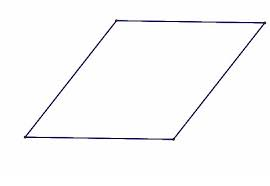The image above is a rhombus.

To compute the area of a rhombus, one essential parameter is needed and this parameter is length of side (a).

The formula for calculating the perimeter of a rhombus:

P = 4a

Where;
P = Perimeter of the rhombus
a = length of side

Let’s solve an example;
Find the perimeter of a rhombus when the length of side is 30 cm.

This implies that;
a = Length of side = 30 cm

P = 4a
P = 4 x 30
P = 120

Therefore, the perimeter of the rhombus is 120 cm.

Calculating the Length of Side using the Perimeter of the Rhombus.

a = P / 4

Where;
a = Length of Side
P = Perimeter of the rhombus

Let’s solve an example;
Given that the perimeter of the rhombus is 180 cm. Find the length of side?

a = P / 4

This implies that;
P = perimeter of the rhombus = 180 cm

a = P / 4
a = 180 / 4
a = 45

Therefore, the length of side is 45 cm.

## How to Calculate and Solve for the Area, Length, Width and Height of a Cuboid | Nickzom Calculator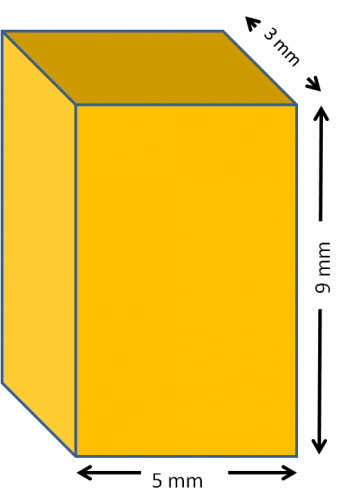The image above represents a cuboid.
To compute the area of a cuboid requires three essential parameters which are the length, width and height of the cuboid.

The formula for computing the area of a cuboid is:

A = 2(lw + lh + wh)

Where:
A = Area of the Cuboid
l = Length of the Cuboid
w = Width of the Cuboid
h = Height of the Cuboid

Let’s solve an example
Find the area of a cuboid with a length of 5 cm, width of 3 cm and a height of 9 cm.

This implies that:
l = length of the cuboid = 5
w = width of the cuboid = 3
h= = height of the cuboid = 9

A = 2(lw + lh + wh)
A = 2(5 x 3 + 5 x 9 + 3 x 9)
A = 2(87)
A = 174

Therefore, the area of the cuboid is 174 cm2.

Calculating the Length of a cuboid when Area, Width and Height are Given

The formula is l = A – 2(w)(h) / 2(w) + 2(h)

Where;
A = Area of the Cuboid
l = Length of the Cuboid
w = Width of the Cuboid
h = Height of the Cuboid

Let’s solve an example:
Find the length of a cuboid with an area of 140 cm2 , a width of 4 cm and a height of 12 cm

This implies that;
A = Area of the cuboid = 140 cm2
w = width of the cuboid = 4 cm
h  = height of the cuboid = 12 cm

l =  A – 2(w)(h) / 2(w) + 2(h)
l = 140 – 2(4)(12) / 2(4) + 2(12)
l = 140 – 96 / 8 + 24
l = 44 / 32
l = 1.375 cm

Therefore, the length of the cuboid is 1.375 cm.

Calculating the Width of a cuboid when Area, Length and Height are Given

The formula is w = A – 2(l)(h) / 2(l) + 2(h)

Where;
A = Area of the Cuboid
l = Length of the Cuboid
w = Width of the Cuboid
h = Height of the Cuboid

Let’s solve an example:
Find the width of a cuboid with an area of 200 cm2 , a length of 5 cm and a height of 12 cm

This implies that;
A = Area of the cuboid = 200 cm2
l = length of the cuboid = 5 cm
h  = height of the cuboid = 12 cm

w =  A – 2(l)(h) / 2(l) + 2(h)
w = 200 – 2(5)(12) / 2(5) + 2(12)
w = 200 – 120 / 10 + 24
w = 80 / 34
w = 2.353 cm

Therefore, the width of the cuboid is 2.353 cm.

Calculating the Height of a cuboid when Area, Length and Width are Given

The formula is h = A – 2(l)(w) / 2(l) + 2(w)

Where;
A = Area of the Cuboid
l = Length of the Cuboid
w = Width of the Cuboid
h = Height of the Cuboid

Let’s solve an example:
Find the height of a cuboid with an area of 300 cm2 , a length of 6 cm and a width of 2 cm

This implies that;
A = Area of the cuboid = 300 cm2
l = length of the cuboid = 6 cm
w  = width of the cuboid = 2 cm

h =  A – 2(l)(w) / 2(l) + 2(w)
h = 300 – 2(6)(2) / 2(6) + 2(2)
h = 300 – 24 / 12 + 4
h = 276 / 16
h = 17.25 cm

Therefore, the height of the cuboid is 17.25 cm.

## How to Calculate and Solve for the Length of the Diagonal and Area of a Rhombus | The Calculator Encyclopedia

The above image is a rhombus.

To compute the area of a rhombus, two essential parameters are needed and this parameters are length of the diagonal (p) and length of the diagonal (q).

The formula for calculating the area of a rhombus:

A = pq ⁄ 2

Where;
A = Area of the rhombus
p = length of the diagonal
q = length of the diagonal

Let’s solve an example;
Find the area of a rhombus when the length of the diagonal (p) is 10 cm and the length of the diagonal (q) is 18 cm.

This implies that;
p = length of the diagonal = 10 cm
q = length of the diagonal = 18 cm

A = pq2
A = 10 x 18 / 2
A = 180 / 2
A = 90

Therefore, the area of the rhombus is 90 cm2.

Calculating the length of the diagonal (p) using Area of the Rhombus and length of the diagonal (q).

p = 2A / q

Where;
A = Area of the rhombus
q = length of the diagonal

Let’s solve an example;
Given that the length of the diagonal (q) is 20 cm with an area of 60 cm2. Find the length of the diagonal (p)?

This implies that;
A = Area of the rhombus = 60 cm2
q = length of the diagonal = 20 cm

p = 2A / q
p = 2 x 60 / 20
p = 120 / 20
p = 6

Therefore, the length of the diagonal is 6 cm.

## How to Calculate and Solve for the Perimeter of a Trapezium | Nickzom Calculator

The image above is a trapezium.

To compute the perimeter of a trapezium, four essential parameters is needed and they are the length of the side (a), length of the side (b), length of the side (c) and length of the side (d).

The formula for calculating the perimeter of a trapezium is;

P = a + b + c + d

Where;

P = Perimeter of the trapezium
a = length of the side
b = length of the side
c = length of the side
d = length of the side

Let’s solve an example;
Find the perimeter of a trapezium with length of side (a) 4 cm, length of side (b) 8 cm, length of side (c) 12, length of side (d) 17 cm.

This implies that;
a = length of side = 4 cm
b = length of side = 8 cm
c = length of side = 12 cm
d = length of side = 17 cm

P = a + b + c + d
P = 4 + 8 + 12 + 17
P = 41

Therefore, the perimeter of the trapezium is 41 cm.

## How to Calculate and Solve for the Volume and Radius of a Sphere | The Calculator Encyclopedia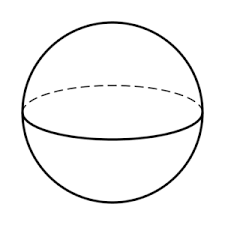The image above is a sphere.

To compute the volume of a sphere, one essential parameter is needed and this parameter is the radius of the sphere (r). You can also use diameter of the sphere (d).

The formula for calculating the volume of a sphere:

V = (4/3)πr³

Where;

V = Volume of the sphere
r = Radius of the sphere

Let’s solve an example:
Find the volume of a sphere when the radius of the sphere is 10 cm.

This implies that;

r = Radius of the sphere = 10 cm

V = (4/3)πr³
V = (4/3)π x (10)³
V = (4/3)π x 1000
V = (4/3)3.142 x 1000
V = (4/3)3142
V = 1.33 x 3142
V = 4188.7

Therefore, the volume of the sphere is 4188.7 cm³.

Calculating the Volume of a Sphere using Diameter of the Sphere.

V = 4πd³ / 24

Where;

V = Volume of the sphere
d = Diameter of the sphere

Let’s solve an example:
Find the volume of a sphere when the diameter of the sphere is 20 cm.

This implies that;

d = Diameter of the sphere = 20 cm

V = 4πd³ / 24
V = 4 x 3.142 x (20)³ / 24
V = 4 x 3.142 x 8000 / 24
V = 100544 / 24
V = 4189.3

Therefore, the volume of the sphere with diameter is 4189.3 cm3.

Calculating the Radius of a Sphere using Volume of the Sphere.

r = 3√(3V / )

Where;

V = Volume of the sphere
r = Radius of the sphere

Let’s solve an example:
Find the radius of a sphere when the volume of the sphere is 250 cm3.

This implies that;

V = Volume of the sphere = 250 cm3

r = 3√(3V / )
r = 3√(3 x 250 / 4 x 3.142)
r = 3√(750 / 12.568)
r = 3√59.675
r = 3.907

Therefore, the radius of the sphere is 3.907 cm.

Calculating the Diameter of a Sphere using Volume of the Sphere.

d = 3√(24V / )

Where;

A = Area of the sphere
d = Diameter of the sphere

Let’s solve an example:
Find the diameter of a sphere when the volume of the sphere is 40 cm3.

This implies that;

V = Volume of the sphere = 40 cm3

d = 3√(24V / )
d = 3√(24 x 40 / 4 x 3.142)
d = 3√(960 / 12.568)
d = 3√76.38
d = 4.24

Therefore, the diameter of the sphere is 4.24 cm.

## How to Calculate and Solve for the Area and Radius of a Sphere | Nickzom Calculator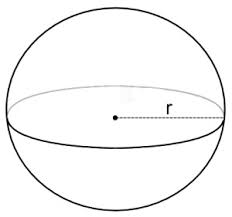The image above is a sphere.

To compute the area of a sphere, one essential parameter is needed and this parameter is the radius of the sphere (r). You can also use diameter of the sphere (d).

The formula for calculating the area of a sphere:

A = 4πr²

Where;

A = Area of the sphere
r = Radius of the sphere

Let’s solve an example:
Find the area of a sphere when the radius of the sphere is 6 cm.

This implies that;

r = Radius of the sphere = 6 cm

A = 4πr²
A = 4 x 3.142 x 6²
A = 4 x 3.142 x 36
A = 452.4

Therefore, the area of the sphere is 452.4 cm2.

Calculating the Area of a sphere using Diameter of the sphere.

A = πd2

Where;

A = Area of the sphere
d = Diameter of the sphere

Let’s solve an example:
Find the area of a sphere when the diameter of the sphere is 8 cm.

This implies that;

d = Diameter of the sphere = 8 cm

A = πd2
A = 3.142 x 82
A = 3.142 x 64
A = 201.08

Therefore, the area of the sphere is 201.08 cm2.

Calculating the Radius of a sphere using Area of the sphere.

r = √(A / )

Where;

A = Area of the sphere
r = Radius of the sphere

Let’s solve an example:
Find the radius of a sphere when the area of the sphere is 22 cm2.

This implies that;

A = Area of the sphere = 22 cm2

r = √(A / )
r = √(22 / 4 x 3.142)
r = √(22 / 12.57)
r = √1.75
r = 1.32

Therefore, the radius of the sphere is 1.32 cm.

## How to Calculate and Solve for the Area, Radius, Diameter and Slant Height of a Cone | The Calculator Encyclopedia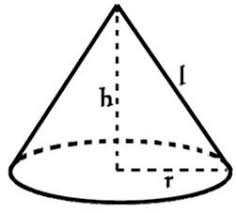The image above is a cone.

To compute the area of a cone, two essential parameters is needed and this parameters are the radius of the cone (r) and the slant height of the cone (h).

The formula for calculating the area of a cone:

A = πrl + πr²

Where;

A = Area of the Cone
r = Radius of the Cone

Let’s solve an example:
Find the area of a cone when the radius of the cone is 9 cm and the slant height of the cone is 12 cm.

This implies that;
r = Radius of the cone = 9 cm
l = Slant height of the cone = 12 cm

A = πrl + πr²
A = 3.142 x 9 x 12 + 3.142 x 9²
A = 339.336 + 254.502
A =  593.83

Therefore, the area of the cone is 593.83 cm².

Calculating the Area of a cone using Diameter and Slant height of the cone.

A = πdl / 2 + πd2 / 4

Where;

d = Diameter of the Cone
l = Slant height of the Cone

Let’s solve an example:
Find the area of a cone when the diameter of the cone is 18 cm and the slant height of the cone is 22 cm?

This implies that;
d = diameter of the cone = 18 cm
l = Slant height of the cone = 22 cm

A = πdl / 2 + πd2 / 4
A = 3.142 x 18 x 22 / 2 + 3.142 (18)2 / 4
A = 1244.232 / 2 + 1018.008 / 4
A = 622.116 + 254.502
A = 876.6

Therefore, the area of the cone with diameter is 876.6 cm2.

Calculating the Slant height of a cone using Radius of the cone and Area of the cone.

l = A – πr2 / πr

Where;

A = Area of the Cone
r = Radius of the Cone

Let’s solve an example:
Find the slant height of a cone when the radius of the cone is 8 cm and the area of the cone is 220 cm2.

This implies that;
A = Area of the cone = 220 cm2
r = Radius of the cone = 8 cm

l = A – πr2 / πr
l = 220 – 3.142 x 82 / 3.142 x 8
l = 220 – 3.142 x 64 / 25.136
l = 220 – 201.088 / 25.136
l = 18.91 / 25.136
l = 0.75

Therefore, the slant height of the cone with radius is 0.75 cm.

## How to Calculate and Solve for the Perimeter or Circumference, Diameter, Radius and Angle of a Sector | The Calculator Encyclopedia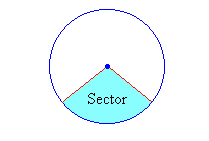The image above is a sector.

To compute the Perimeter or Circumference a sector, two essential parameters is needed and this parameters are the radius of the sector (r) and the angle of the sector (θ). You can also use the diameter of the sector (d).

The formula for calculating the Perimeter or Circumference of a sector:

P = 2r + θ / 360(2πr)

Where;

P = Perimeter or Circumference
r = Radius of the sector
θ = Angle of the Sector

Let’s solve an example:
Find the perimeter or circumference of a sector when the radius of the sector is 14 cm and the angle of the sector is 60°

This implies that;

r = Radius of the sector = 14 cm
θ = Angle of the sector = 60°

P = 2r + θ / 360(2πr)
P = 2 x 14 + 60 / 360(2 x 3.142 x 14)
P = 28 + 0.1667 (87.97)
P = 28 + 14.66
P = 42.66

Therefore, the perimeter or circumference of the sector is 42.66 cm.

Calculating the Perimeter or Circumference of a Sector using Diameter and Angle of the sector.

P = d + θ / 360(πd)

θ = Angle of the sector
d = Diameter of the sector

Let’s solve an example;
Find the perimeter or circumference of a sector when the diameter of the sector is 20 cm and the angle of the sector is 80°.

This implies that;

d = Diameter of the sector = 20 cm
θ = Angle of the sector = 80°

## How to Calculate and Solve for the Area, Radius, Diameter and Angle of a Sector | The Calculator Encyclopedia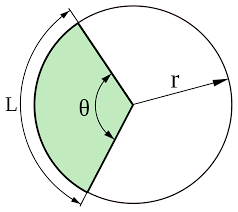The image above is a sector.

To compute the area of a sector, two essential parameters is needed and this parameters are the radius of the sector (r) and the angle of the sector (θ). You can also use the diameter of the sector (d).

The formula for calculating the area of a sector:

Area of a sector = (θ/360)[πr²]

Where;

A = Area of the Sector
r = Radius of the Sector
θ = Angle of the Sector

Let’s solve an example:
Find the area of a sector when the radius of the sector is 7 cm and the angle of the sector is 9°

This implies that;

r = Radius of the sector = 7 cm
θ = Angle of the sector = 9°

A = θ / 360 x πr2
A = (9/360)[π x 7²]
A = 0.025 x π x 49
A = 3.848

Therefore, the area of the sector is 3.848 cm2.

Calculating the Area of a Sector using Diameter and Angle of the sector.

The formula is A = θ / 360 x πd2 / 4

Where;

θ = Angle of the sector
d = Diameter of the sector

Let’s solve an example;
Find the Area of a sector when the diameter of the sector is 12 cm and the angle of the sector is 18°.

This implies that;

d = Diameter of the sector = 12 cm
θ = Angle of the sector = 18°

A = θ / 360 x πd2 / 4
A = 18 / 360 x 3.142 (144) / 4
A = 18 / 360 x 452.448 / 4
A= 18 / 360 x 113.112
A= 0.05 x 113.112
A = 5.656

Therefore, the area of the sector with diameter is 5.656 cm

How to Calculate Angle of a Sector when Area of the Sector and Radius of the Sector is Given

θ = 360 (A) / πr2

where;

r = Radius of a sector
A = Area of a sector

Let’s solve an example;
Given that the area of a sector is 15 cm2 and the radius of the sector is 5 cm. Find the angle of the sector?

This implies that;
A = Area of the sector = 15 cm2
r = Radius of the sector = 5 cm

θ = 360 (A) / πr2
θ = 360 (15) / 3.142 (5)2
θ = 5400 / 3.142 (25)
θ = 5400 / 78.55
θ = 68.746

Therefore, the angle of the sector is 68.746°.

How to Calculate Angle of a Sector when Area of the Sector and Diameter of the Sector is Given

θ = 1440 (A) / πd2

where;

d = Diameter of a sector
A = Area of a sector

Let’s solve an example;
Given that the area of a sector is 22 cm2 and the diameter of the sector is 10 cm. Find the angle of the sector?

This implies that;
A = Area of the sector = 22 cm2
d = Diameter of the sector = 10 cm

θ = 1440 (A) / πr2
θ = 1440 (22) / 3.142 (10)2
θ = 31680 / 3.142 (100)
θ = 31680 / 314.2
θ = 100.88

Therefore, the angle of the sector is 100.88°.

How to Calculate Diameter of a Sector when Area of the Sector and Angle of the Sector is Given

d = √1440 (A) / πθ

where;

θ = Angle of a sector
A = Area of a sector

Let’s solve an example;
Given that the area of a sector is 24 cm2 and the angle of the sector is 10°. Find the diameter of the sector?

This implies that;
A = Area of the sector = 24 cm2
θ = Angle of the sector = 10°

d = √1440 (A) / πθ
d = √1440 (24) / 3.142 x 10
d = √34560 / 31.42
d = √1099.936
d = 33.165

Therefore, the diameter of the sector is 33.165 cm.

How to Calculate Radius of a Sector when Area of the Sector and Angle of the Sector is Given

r = √360 (A) / πθ

where;

θ = Angle of a sector
A = Area of a sector